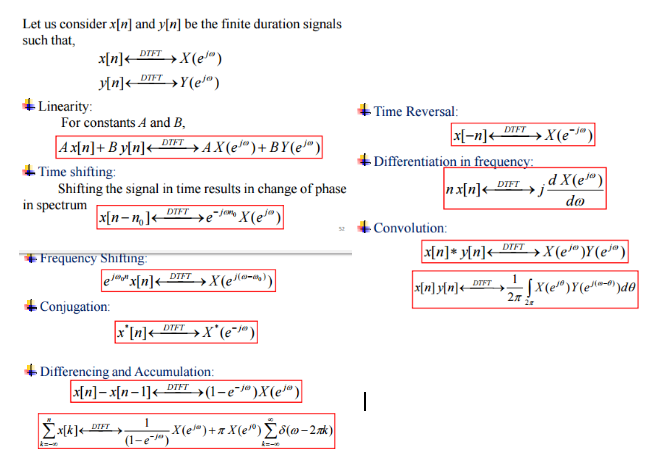### Notes of Digital Signal Analysis and Processing [CT 704]

#### Discrete Time Signal and System

Discrete Time Signal

Introduction

- Signal is something that convey some information about state and behaviour of the physical system.
- Mathematically, signals are represented as a function of one or more independent variables.
- Signal processing deals with representation, transformation and manipulation of signals and the information contained in those signals.
- Signal processing is generally used to obtain an output signal from an input signal. Also, it is used for signal interpretation like in speech recognition system.
- Digital signal processing is based on the processing sequences of the samples.
- In digital signal processing, the signals are represented by sequences of finite precision numbers and digital computation is done for processing of those signals.
- In continuous time signals, there are infinite possible values between start and end time.
- In discrete time signals, the possible values of each element is finite and can be represented with computer bits (i.e. 0 or 1).
- Continuous time signal must be made discrete when brought to a computer system for processing or manipulation or representation.

Why digital signal is prefered over continuous signal?

- Digital signals are immune to the imperfections of real electronic appliances which tend to spoil analog signals.
- In digital signals, information is determined by presence or absence of data bits, so they have greater noise immunity. But, analog signals varies continuously so are affected by all levels of noise.
- Digital signals can be processed by the digital circuitry, which is comparatively cheaper.
- Digital signals uses less bandwidth. So, many informations can be crammed into same space.
- Digital signals can be encrypted that provides high level of security.
- Digital signals can be transmitted over a long distance with minimum errors.
- Electromagnetic interferences are less in digital signals.

Discrete Time Processing of Continuous Signals

Sampling of Continuous Time Signals:
- For discrete time processing, firstly the continuous time signals are sampled to construct a discrete time signal.
- Sampling is done by taking an interval called sampling interval (T) such that:
y(n) = x(nT) for all n belongs to integers.
Where, y(n) is the sampling output of time n.
x(nT) is the corresponding value of input signal at time nT.

Reconstruction:
- After necessary processing of discrete time signals, it must be converted back to continuous time signals, which is done by reconstruction using interpolation methods.

Practical Considerations while Reconstruction:
1. Zero-order hold : The value of each sample y(n) is held constant for duration T, so that x(t) = y(n) for time duration t = nT to t = (n+1)T.
2. Linear Interpolation : The straight line is used to connect two alternate samples.
3. Ideal Interpolation : It results in smooth curve passing through the samples.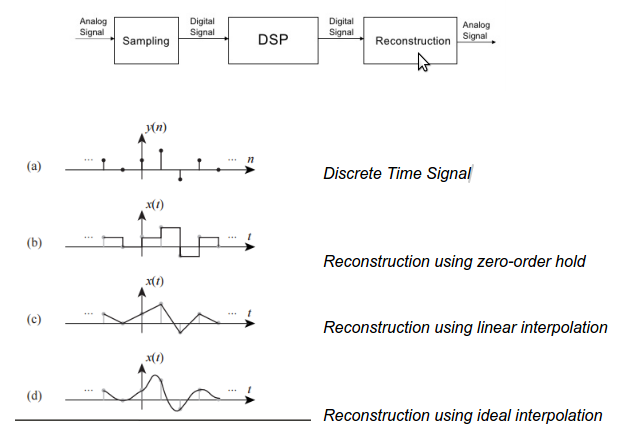Discrete Time Signals

- Discrete time signals are those signals in which the signal has values only for discrete values of time.
- Discrete time signals can be obtained by sampling of continuous time signals or by accumulating variables over a time.

- It is represented as:
1. Sequence of numbers : x[n] = {1,0.8,0.5,0.685}
2. Function of n : x[n] = 0.8n for n >= 0
0 otherwise
3. Graph :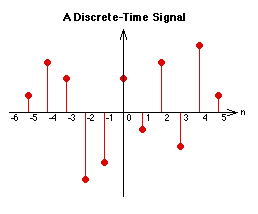Discrete Time Unit Step Signal

- It models the phenomenon that changes value in steps.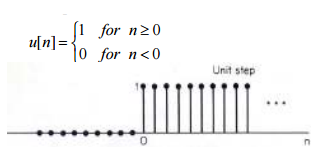Discrete Time Unit Impulse Signal

- It models the phenomenon that appear only for short period of time.
- Any discrete time signals can be expressed in terms of impulses as shown in figure: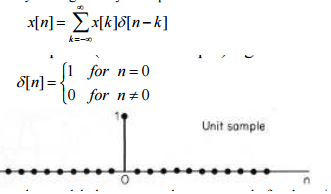Discrete Time Exponential Signal

- It may be real or complex.
- It may be growing or decaying.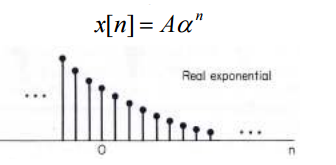Discrete Time Sinusoidal Signal

- In case of Discrete time sinusoidal signal,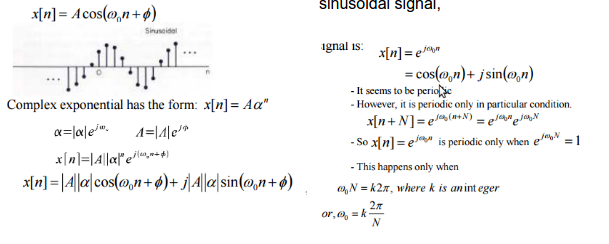Energy and Power Signal

- The discrete time signals that have finite total energy but zero average power are called energy signals.
- The discrete time signals that have finite average power but infinite total energy are called power signals.
- Generally, periodic signals are power signals and aperiodic finite signals are energy signals.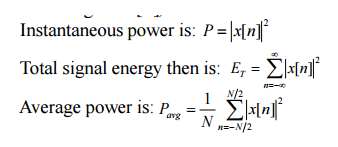Q) Check signal x[n] = u[n] and x[n] = δ[n] is energy or power signal.

```1) For x[n] = u[n]
We know that:
x[n] = u[n] = 1 ; n >= 1
= 0 ; n < 0
Now,
Average power is calculated as:
Pavg = limk-->∞ [1/(2k +1)] ∑n=0 to ∞ u2[n]
= limk-->∞ [(k + 1) / (2k + 1)]
= 1/2```

Total energy is calculated as:
E(t) = ∑n=-∞ to ∞ | x[n] |2
= ∞

Hence, x[n] = u[n] is a power signal.

```2) For x[n] = δ[n]
We know that:
x[n] = δ[n] = 1 ; n= 0
= 0 ; otherwise
Now,```

Total energy is calculated as:
E(t) = ∑n=-∞ to ∞ | x[n] |2
= 0 + 1 + 0
= 1

Average power is calculated as:
Pavg = limk-->∞ [1/(2k +1)] ∑n=0 to ∞ u2[n]
= limk-->∞ [1 / (2k + 1)]
= 1/∞
= 0

Hence, x[n] = δ[n] is a energy signal.

Periodicity of Discrete Signal

- A discrete time signal is said to be periodic if it repeats itself after every fixed samples.
- Mathematically, a discrete time signal is said to be periodic if and only if given condition is true:
x[n + N] = x[n] for all n
- The smallest value on N for which the condition holds is called fundamental period.
- The fundamental frequency is given by : f = 1 / N
- The fundamental radian frequency is given by

Q) Check the signal x[n] = cos(2nπ/5) + sin(nπ/3) is periodic or not.

```The given signal is:
x[n] = cos(2nπ/5) + sin(nπ/3)```

A signal is said to be periodic if the frequency is rational such that : fo = r / N.
where, r and N both are integers.
N is the fundamental period of the signal.

Now,
x[n] = cos(2nπ/5) + sin(nπ/3)

Here,
cos(2nπ/5) = cos(2n . 1/5 . π) = cos(2π . 6/30 . n)
sin(nπ/3) = sin(2n . 1/6 . π) = sin(2π. 5/30 . n)

As the frequency of both components can be expressed in the form fo = r / N with N = 30,
the given signal is periodic with period N = 30.

Q) Determine which signals are periodic and compute fundamental period.

1. cos(πn2/8)

```The given signal is:
cos(πn2/8)
= cos(π . 1/8 .n2)```

The frequency is a rational number given by:
fo = r / N = 1 / 8

Hence, the given signal is periodic with fundamental period N = 8.

2. cos(π/2) cos(πn/4)

```The given signal is:
cos(n/2) cos(πn/4)
= cos(n/2 - πn/4) + cos(n/2 + πn/4)
= cos(n/4 . (2-π)) + cos(n/4 . (2+π))```

The frequency can not be a rational number.
Hence, the given signal is not a periodic signal.

Odd and Even Parts of a Function

- Let us consider for a function f(n);
E(n) represents the even part and O(n) represents the odd part.

- The following must be true:
1. f(n) = E(n) + O(n)
2. f(-n) = E(-n) + O(-n) = E(n) - O(n)

- So, we can obtain:
E(n) = { f(n) + f(-n) } / 2
O(n) = { f(n) - f(-n) } / 2

Q) Find even and odd part of the signal given below:

```The given signal is:
x(n) = 1 for -4 <= n <= 0
= 0 for 1 <= n <= 4```

Now,
For -4 <= n <= 0;
E(n) = { x(n) + x(-n) } / 2 = (1+2)/2 = 3/2
O(n) = { x(n) - x(-n) } / 2 = (1-2)/2 = - 1/2

For n = 0;
E(n) = { x(n) + x(-n) } / 2 = (1+1)/2 = 1
O(n) = { x(n) - x(-n) } / 2 = (1-1)/2 = 0

For 1 <= n <= 4;
E(n) = { x(n) + x(-n) } / 2 = (2+1)/2 = 3/2
O(n) = { x(n) - x(-n) } / 2 = (2-1)/2 = 1/2

Hence,
E(n) = 3/2 for -4 <= n <= -1
= 1 for n = 0
= 3/2 for 1 <= n <= 4

O(n) = - 1/2 for -4 <= n <= -1
= 0 for n = 0
= 1/2 for 1 <= n <= 4

Transformation of Independent Variable

Time Shifting

- It is shifting of a signal in time.
- It is done by adding or subtracting the amount of shift of the independent variable (n) in the function.
- Subtracting will shift the signal to right. It is called time delay.
- Adding will shift the signal to left. It is called time advance.

Time Scaling

- It compresses and dilates the signal.
- It is done by multiplying the independent variable (n) by scale amount.
- If amount > 1, the signal becomes narrower. It is called compression.
- If amount < 1, the signal becomes wider. It is called dilation.

Time Reversal

- It flips the signal over y-axis.
- It is done by scaling the signal with negative amount.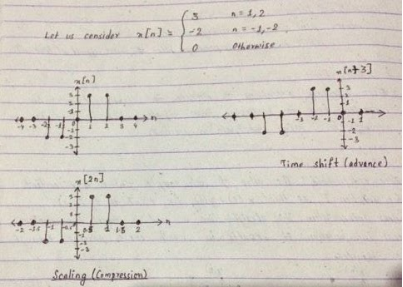Discrete time fourier series and properties

- Fourier series helps to represent and analyze the discrete time signals in frequency domain.
- The frequency range of discrete time signal is unique over the interval (-

Basic Derivation

Let us consider a periodic sequence x(n) with period N. It means x(n) = x(n+N) for all integers n.
The fourier series representation for x(n) consists of N harmonically related exponential functions.
ej2kn/N where k = 0, 1, 2, ………………. N-1
And is expressed as:
x(n) = ∑ (ckej2kn/N) from k = 0 to k = N-1 (4.2.1)
Where {ck} are coefficients of fourier series representation of periodic discrete time signals.

Derivation of Fourier Coefficients

The derivation is as follows: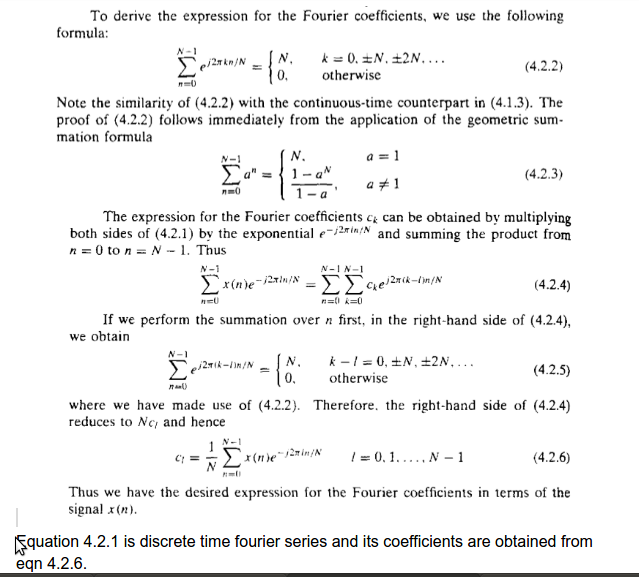Properties:

The properties of fourier series are as follows: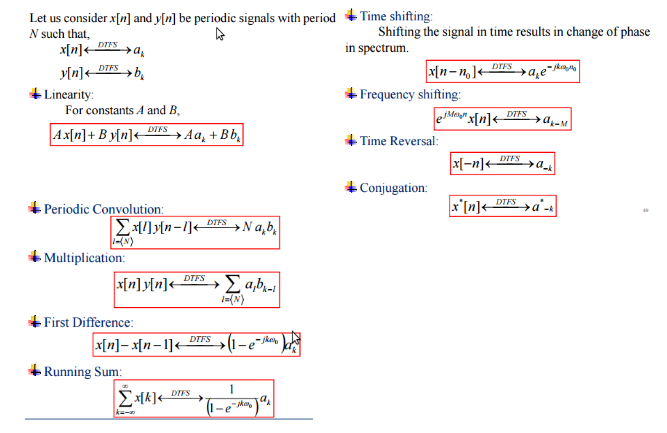Discrete time fourier transform and properties

- The frequency range of fourier transform of aperiodic discrete time signals is unique over the frequency interval of (-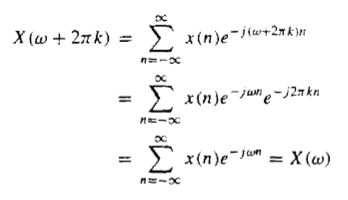Properties:

The properties of fourier transform are as follows: